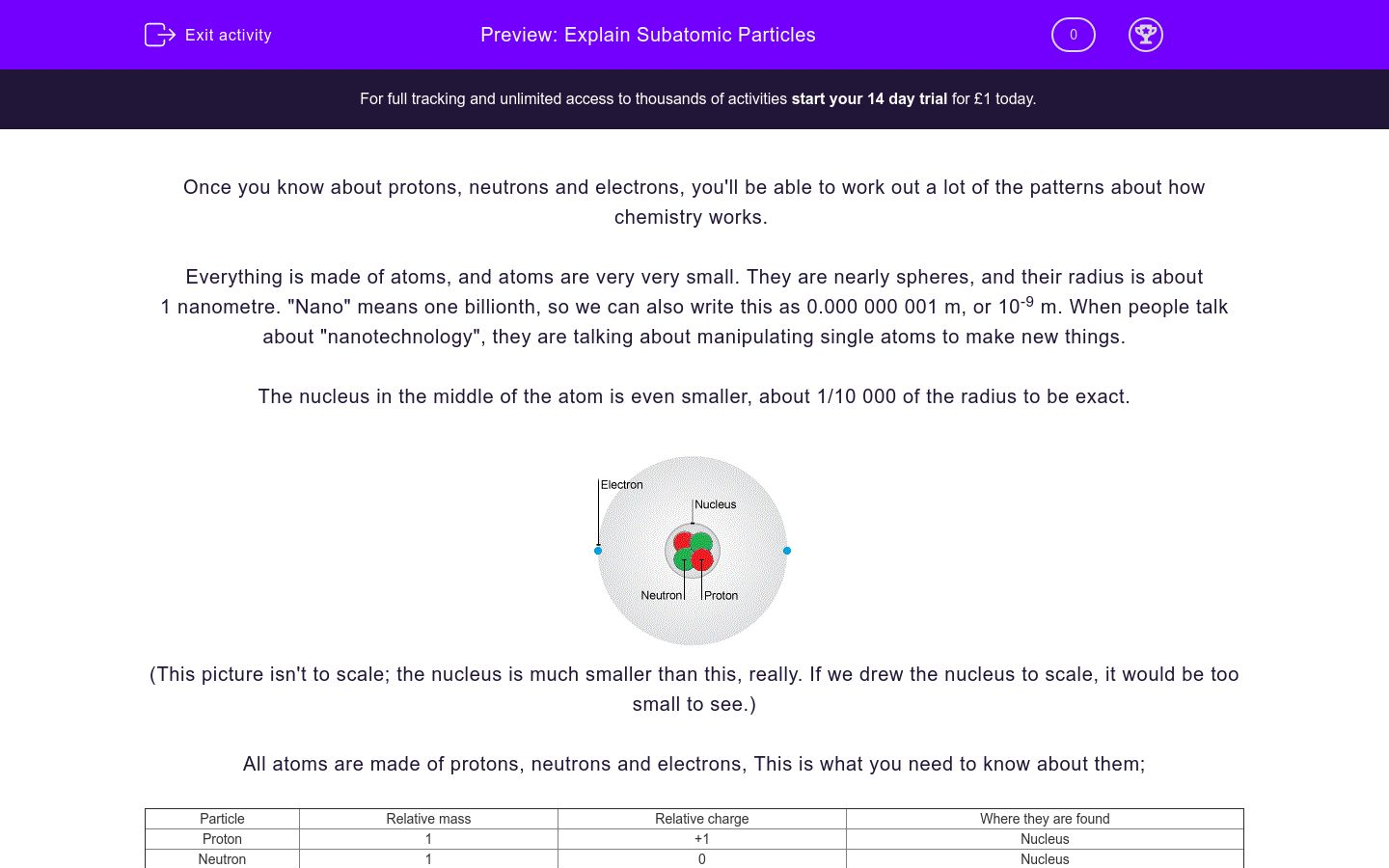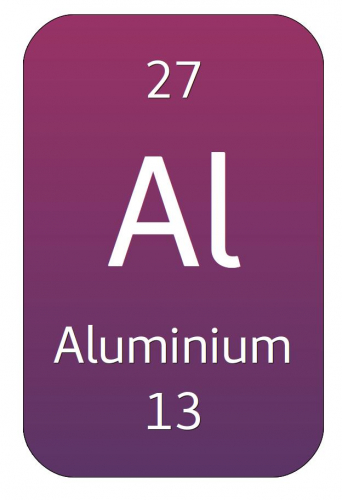# Explain Subatomic Particles

In this worksheet, students will learn about protons, neutrons and electrons, and the way they join together to make atoms.Key stage:  KS 4

Difficulty level:### QUESTION 1 of 10

Once you know about protons, neutrons and electrons, you'll be able to work out a lot of the patterns about how chemistry works.

Everything is made of atoms, and atoms are very very small. They are nearly spheres, and their radius is about 1 nanometre. "Nano" means one billionth, so we can also write this as 0.000 000 001 m, or 10-9 m. When people talk about "nanotechnology", they are talking about manipulating single atoms to make new things.

The nucleus in the middle of the atom is even smaller, about 1/10 000 of the radius to be exact.(This picture isn't to scale; the nucleus is much smaller than this, really. If we drew the nucleus to scale, it would be too small to see.)

All atoms are made of protons, neutrons and electrons, This is what you need to know about them;

 Particle Relative mass Relative charge Where they are found Proton 1 +1 Nucleus Neutron 1 0 Nucleus Electron 0.0005 -1 Shells

(Remember: Protons are positive, neutrons are neutral).

If we want to know what an atom is like, we need to know how many protons, neutrons and electrons it has. There are two terms you need to know:

The atomic number of an element is how many protons there are in the nucleus.

The mass number of an element is the total number of protons and neutrons in the nucleus. We don't count electrons, because they are much lighter.

When you look up an element in the periodic table, it will tell you the  atomic number and mass number, like this:Different periodic tables put the numbers in different places, so remember that the larger number is the mass number, and the smaller number is the atomic number. It's also a good idea to get a copy of the version of the periodic table your exam board uses, so you can get used to it.

To work out the numbers of protons, neutrons and electrons, follow these rules:

The number of protons is the same as the atomic number.

So sodium has 11 protons

The number of neutrons is the difference between the mass number and the atomic number.

So the number of neutrons in sodium = 23 - 11 = 12 neutrons

The number of electrons in an atom is the same as the number of protons. Atoms are uncharged, so the number of +1 charged protons has to be the same as the number of -1 charged electrons.

So sodium atoms have 11 electrons

Once you've done some for yourself, it becomes easy to talk about atomic numbers and mass numbers. All you need to do is adding and subtracting.

Match up these half-sentences about the size of atoms and the nucleus.

## Column B

Atoms are small, their typical radius is
one nanometre.
"Nano" means
one billionth, or 10-9.
The nucleus is even smaller than an atom; its radi...
10-13 m.
This means that the radius of a nucleus is
ten thousand times smaller.

Complete the gaps in this paragraph about neutrons and protons. Use these words:

charges

neutron

nucleus

1

proton

## Column B

Atoms are small, their typical radius is
one nanometre.
"Nano" means
one billionth, or 10-9.
The nucleus is even smaller than an atom; its radi...
10-13 m.
This means that the radius of a nucleus is
ten thousand times smaller.

What are the properties of an electron?

## Column B

Atoms are small, their typical radius is
one nanometre.
"Nano" means
one billionth, or 10-9.
The nucleus is even smaller than an atom; its radi...
10-13 m.
This means that the radius of a nucleus is
ten thousand times smaller.

Match these phrases with their definitions.

## Column B

Atomic number
Total number of protons and neutrons in the nucleu...
Mass number
Central part of the atom, containing protons and n...
Nucleus
Number of protons in the nucleus

The atomic number of fluorine is 9, and the mass number is 19. Which of these statements are true?

Fluorine has 9 protons.

Fluorine has 9 neutrons.

Fluorine has 10 protons.

Fluorine has 10 neutrons.

Fluorine has 19 protons.

Fluorine has 19 neutrons.

The periodic table box for aluminium looks like this:Match up these half-sentences about aluminium.

## Column B

The atomic number is
27, because that's the larger number
The mass number is
14, because that's the difference between the mass...
The number of neutrons is
13, because that's the smaller number

The box in the periodic table for lithium looks like this:Use this information to complete the description of the lithium atom.

## Column B

The atomic number is
27, because that's the larger number
The mass number is
14, because that's the difference between the mass...
The number of neutrons is
13, because that's the smaller number

The periodic table box for potassium is:A student wrote these sentences about potassium. Mark their work by ticking the sentences they got right.

Potassium has 19 protons, because the atomic number is 19.

Potassium has 39 neutrons, because the mass number is 39.

The electron number is the same as the atomic number.

We get the mass number by adding protons and neutrons.

We don't include electrons in the mass, because they have no mass.

Look up phosphorus in a periodic table. Use the information to fill in the gaps in this paragraph about phosphorus. Type in numbers, not words.

Potassium has 19 protons, because the atomic number is 19.

Potassium has 39 neutrons, because the mass number is 39.

The electron number is the same as the atomic number.

We get the mass number by adding protons and neutrons.

We don't include electrons in the mass, because they have no mass.

Pick a column of the periodic table.  It doesn't matter which one. Work out the number of protons and neutrons for each element. Which of these sentences is true?

Proton number increases faster than neutron number

Neutron number increases faster than proton number

Proton number and neutron number both increase equally

There is no pattern

• Question 1

Match up these half-sentences about the size of atoms and the nucleus.

## Column B

Atoms are small, their typical ra...
one nanometre.
"Nano" means
one billionth, or 10-9...
The nucleus is even smaller than ...
ten thousand times smaller.
This means that the radius of a n...
10-13 m.
EDDIE SAYS
You will learn a lot about prefixes like "nano", and notation like 10-9 in GCSE maths and physics. They're really useful for describing very small things, like atoms.
• Question 2

Complete the gaps in this paragraph about neutrons and protons. Use these words:

charges

neutron

nucleus

1

proton

EDDIE SAYS
Remember- protons are positive. Protons and neutrons both have a relative mass of 1.
• Question 3

What are the properties of an electron?

EDDIE SAYS
Check the table in the introduction if you're not sure. The table of properties is important to learn by heart.
• Question 4

Match these phrases with their definitions.

## Column B

Atomic number
Number of protons in the nucleus
Mass number
Total number of protons and neutr...
Nucleus
Central part of the atom, contain...
EDDIE SAYS
You might be asked about these definitions in an exam, so take care to get them the right way round. As you do some calculations, it will help you remember which way round they go.
• Question 5

The atomic number of fluorine is 9, and the mass number is 19. Which of these statements are true?

Fluorine has 9 protons.
Fluorine has 10 neutrons.
EDDIE SAYS
The atomic number is the number of protons, so you don't need to do any addition or subtraction. To get the number of neutrons, subtract the atomic number from the mass number; 19 - 9 = 10.
• Question 6

The periodic table box for aluminium looks like this:Match up these half-sentences about aluminium.

## Column B

The atomic number is
13, because that's the smaller nu...
The mass number is
27, because that's the larger num...
The number of neutrons is
14, because that's the difference...
EDDIE SAYS
Most printed periodic tables tell you which number is the atomic number and which is the mass number. It's much better to think "mass number is larger, atomic number is smaller", because then it doesn't matter what the printed layout is.
• Question 7

The box in the periodic table for lithium looks like this:Use this information to complete the description of the lithium atom.

EDDIE SAYS
There's a lot going on here, so don't worry if you found it harder. There are two numbers for each element- the larger one is the mass number, and the smaller one is the atomic number. The atomic number gives you both the protons and the electrons. To get the neutron number, subtract atomic number from mass number.
• Question 8

The periodic table box for potassium is:A student wrote these sentences about potassium. Mark their work by ticking the sentences they got right.

Potassium has 19 protons, because the atomic number is 19.
The electron number is the same as the atomic number.
We get the mass number by adding protons and neutrons.
EDDIE SAYS
They got a lot of things right, but they made two mistakes. The mass number isn't the number of neutrons; to get the neutron number, you need to subtract the atomic number from the mass number. Electrons do have some mass, but not enough to affect the overall mass very much.
• Question 9

Look up phosphorus in a periodic table. Use the information to fill in the gaps in this paragraph about phosphorus. Type in numbers, not words.

EDDIE SAYS
There are two important things to remember in questions like this. Of the two numbers for each element, the mass number is larger, and the atomic number is smaller. Once you have the mass and atomic numbers, the number of neutrons is the difference between the two.
• Question 10

Pick a column of the periodic table.  It doesn't matter which one. Work out the number of protons and neutrons for each element. Which of these sentences is true?

Neutron number increases faster than proton number
EDDIE SAYS
As atoms get heavier, the protons push each other apart, because they are all positively charged. You need more and more neutrons to stop this happening.
---- OR ----

Sign up for a £1 trial so you can track and measure your child's progress on this activity.

### What is EdPlace?

We're your National Curriculum aligned online education content provider helping each child succeed in English, maths and science from year 1 to GCSE. With an EdPlace account you’ll be able to track and measure progress, helping each child achieve their best. We build confidence and attainment by personalising each child’s learning at a level that suits them.

Get started